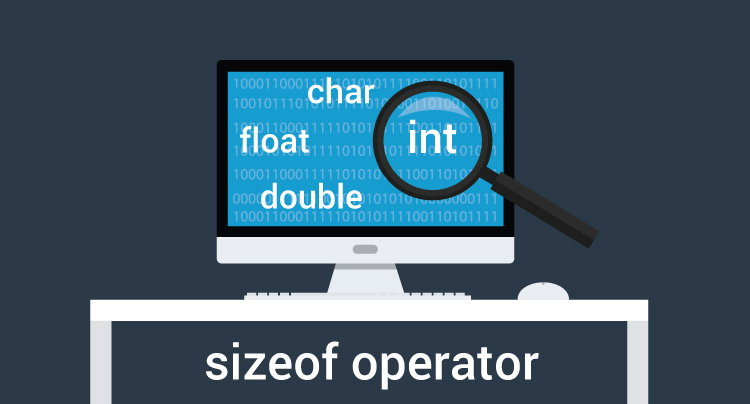# C Program to Find the Size of int, float, double and char

In this example, you will learn to evaluate the size of each variable using sizeof operator.To understand this example, you should have the knowledge of the following C programming topics:

## Program to Find the Size of a variable

``````#include<stdio.h>
int main() {
int intType;
float floatType;
double doubleType;
char charType;

// sizeof evaluates the size of a variable
printf("Size of int: %ld bytes\n", sizeof( intType ));
printf("Size of float: %ld bytes\n", sizeof( floatType ));
printf("Size of double: %ld bytes\n", sizeof( doubleType ));
printf("Size of char: %ld byte\n", sizeof( charType ));

return 0;
}

``````

Output

```Size of int: 4 bytes
Size of float: 4 bytes
Size of double: 8 bytes
Size of char: 1 byte
```

In this program, 4 variables intType, floatType, doubleType and charType are declared having `int`, `float`, `double` and `char` type respectively.

Then, the size of each variable is computed using the `sizeof` operator.